Worksheet 3. Lorentz force
Оценка 4.7

# Worksheet 3. Lorentz force

Оценка 4.7
docx
08.05.2020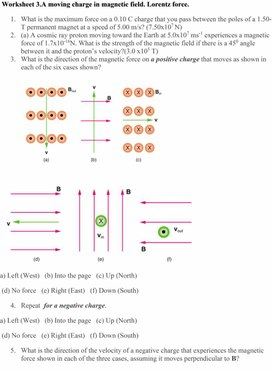Worksheet 3. Lorentz force.docx

Worksheet 3.A moving charge in magnetic field. Lorentz force.

1.      What is the maximum force on a 0.10 C charge that you pass between the poles of a 1.50-T permanent magnet at a speed of 5.00 m/s? (7.50x107 N)

2.      (a) A cosmic ray proton moving toward the Earth at 5.0x107 ms-1 experiences a magnetic force of 1.7x10-16N. What is the strength of the magnetic field if there is a 450 angle between it and the proton’s velocity?(3.0 x105 T)

3.      What is the direction of the magnetic force on a positive charge that moves as shown in each of the six cases shown?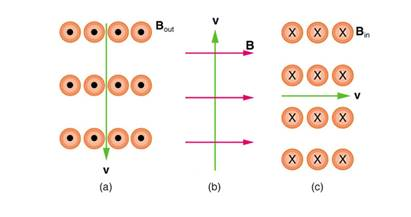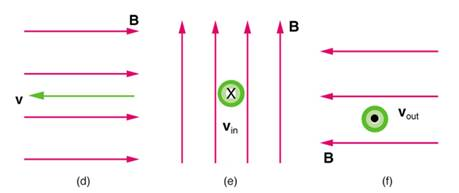a) Left (West)   (b) Into the page   (c) Up (North)

(d) No force   (e) Right (East)   (f) Down (South)

4.      Repeat  for a negative charge.

a) Left (West)   (b) Into the page   (c) Up (North)

(d) No force   (e) Right (East)   (f) Down (South)

5.      What is the direction of the velocity of a negative charge that experiences the magnetic force shown in each of the three cases, assuming it moves perpendicular to B?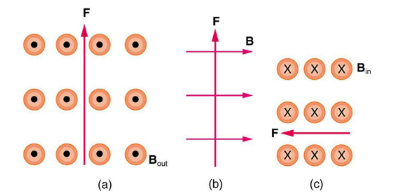a) East (right)    (b) Into page   (c) South (down)

6.      What is the direction of the magnetic field that produces the magnetic force on a positive charge as shown in each of the three cases in the figure below, assuming  B is perpendicular to v?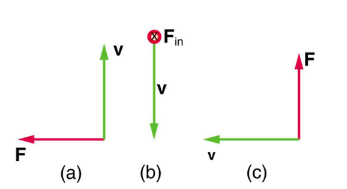(a) Into page     (b) West (left)    (c) Out of page

Скачать файл